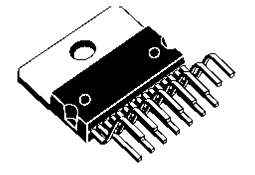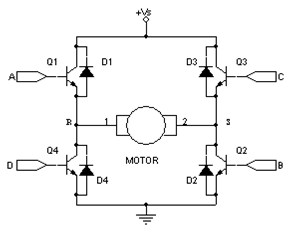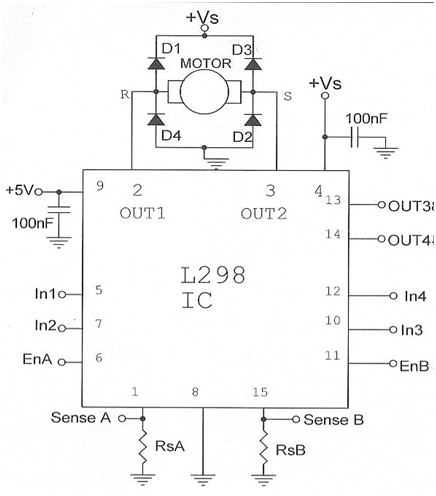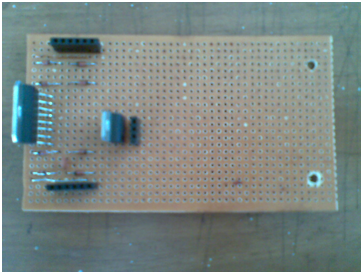## Wednesday, July 30, 2014

### METAL DETECTOR

Hello again.. This time I’m going to tell you how to make a very simple metal detector with a minimum cost. I made this with a group for a module of my degree program. The basic circuit we took from the internet and did some modifications according to my requirements.  For this we needed few devices as below.

NE555
47kΩ resister
2.2 μF, 3.3 μF, 10 μF capacitors
Speaker (8Ω resistance)
Copper wires of 0.3mm in diameter
9V battery
Switch
Connecting wires

Here we used a 555 IC and it gives accurate oscillations and these oscillations are controlled by the capacitor network and inductor used in the circuit. When the inductor goes near the metal the inductance change and frequency varies according to that. Therefore when the inductor meets a metal it gives out a different sound from the speaker.

This is the basic circuit for the metal detector and we changed few capacitor values from the original circuit.

FIGURE 1: BASIC CIRCUIT

All the components like resisters, speaker, capacitors and IC can be obtained easily but when comes to the inductor it becomes more complex. When making the inductor we used copper wires with 0.3mm diameter. Then we wound the copper wire around circular rig foam for 200 turns. This rig foam circle has a diameter of 15cm and height of 1.5 cm. We got these values why doing few calculations and tests.

To find a suitable diameter for the inductor we used inductors with few different measurements and connected it to the above circuit and checked the accuracy by measuring output frequency. We found that with the increment of the diameter the accuracy also increasing. So we selected 15cm. Then we removed an inner circular portion from the rig form to make it air core and by using equation 1 below, we calculated the number of turns of the inductor.

L – Inductance
D – Diameter
N – Number of turns
H- Height

According to the circuit we could get 10mH inductance by 187 turns. But for better inductance we used 200 turns which gives an inductance of 11.5mH.

FIGURE 2: INDUCTOR

After making the inductor the circuit in Figure 1 can be connected to the project board and test it. We did not use PCB because this is a simple circuit. We made the circuit on a dot board as below.

FIGURE 3: CIRCUIT MOUNTED TO THE DOT BOARD

Now the basic metal detector is ready. To give a better finishing for the device we made a box with Perspex and attached the circuit on the dot board, batteries, switch  and speaker to that box.

FIGURE 4: COMPONENTS FIXED TO THE PERSPEX BOX

Then we connected this box to the wood handle of the detector and then we connected the inductor to a plastic plate and that plastic plate connected to a rod.

FIGURE 4: PERSPEX BOX CONNECTED TO THE ROD

FIGURE 5: completed metal detector

This is a very simple metal detector and this can be improved in many aspects like technically, appearance etc.  Anyway from this post you can get the fundamental understanding about  a metal detector and let’s meet with another post soon. Thank you for reading..

Special thanx  to Sampath and Amila...

## Saturday, March 22, 2014

### LINE FOLLOWING ROBOT -III

Hello again.. so far we discussed about the sensor panel and the motor drive system of the line follower.. In order to finalize this, we need to program it and finish the structure.. From this article I will explain about the control system and the structure..

Controller

The earlier connected sensor panel and the motor drive system should be connected in order to make the robot work according to the senses received by the sensors. That part is done with the use of Arduino Board. The sensor arrays are connected to the arduino through analog pins and sensor panel gets power to its operation from the arduino (52 port).

Figure 1- The Arduino Board

According to the sensor values received, the arduino is programmed to pass a PWM value to In1, in2, IN3 and In4.This PWM value allows to control the speed of the motors.

PWM (Pulse With Modulation ) :

PWM technology is used to control  the speed of motors. Here the process of varying the width of voltage pulses takes place.

Figure 2- PWM Waveforms

According to the figure above, it is clear that by varying the existing time of high (12V) and low(0V) voltages, the average voltage of each instance can be changed. Therefore the speed can be changed. Here the maximum PWM value is 255 and minimum is 0. By assigning any value in between these to values, the rotation speed of the motors can be controlled.

Structure

The structure is done with Perspex .This has 3 wheels where two main wheels are directly connected to the 2 motors. The 3rd wheel is at back . At the front , the sensor panel is connected closer to the ground.

Figure 3 - The final structure without circuits

Figure  4 –The Final Line Following Robot

I have not given the arduino coding or the program for the bot.  It is because the code is something to think and do..I hope you will be able to do the correct coding and complete the simple line following robot..

Now I think you got a basic understanding about the line follower and Thank you very much for reading..

Special Thanx to Vibhatha, Lakshan, Anuradha and Prabhodi

## Monday, November 11, 2013

### LINE FOLLOWING ROBOT - II

THE MOTOR DRIVE SYSTEM

Hello again. Earlier we discussed about the sensor panel of the line following bot and from this article I'm going to explain about the motor drive system of this bot.

Here the motor drive system is based on the L298 IC.  L298 IC is a high voltage, high current dual  full-bridge driver. Two separate motors can be connected to these two H-Bridges.Figure 1 - L298 IC                                              Figure 2– H- BridgeFigure 3- Motor Drive Circuit connected to L298 IC

Here there are two separate input couples for the two motors. And along with the inputs, there are two enable pins to operate the two motors.  Two  enable inputs are provided to enable or disable the device independently of the input signals. i.e. even though  In1 and In2 receive logic ‘1’, the motor still does not operate until EnA enable pin receives a logic ‘1’.

Table1 – Motor Behavior according to the given inputs

 Motor Behavior EnA = 1 In1 = 1    In2 = 0 In1 = 0    In2 = 1 In1 = In2 Turn to Right Turn to Left Sudden Stop EnA = 0 In1 = 1 or 0 In2 = 1 or 0 The motor rotates freely or stay at rest

Here In1,In2 and EnA  inputs are to control motor 1. The motor 2 is controlled by In3,In4 and EnB. Two outputs from Pin2 and Pin3 of the IC are taken to connect motor1 and motor 2 is connected to the outputs taken from Pin 13 and Pin 14. When connecting these outputs taken from the IC to the motor, D1, D2, D3 and D4 free willing diodes also should be connected to the motor. For this purpose fast recovery diodes should be selected. Here we have used 1N4148 diodes.Figure 4 –The Final Motor Driver Circuit

From the female ports included in the circuit as shown, the two motors and inputs can be connected.

So I guess you got some idea about the motor drive system and thank you for reading.